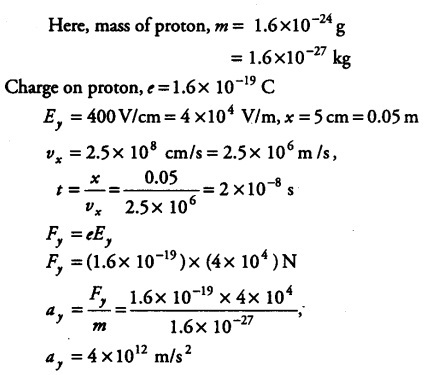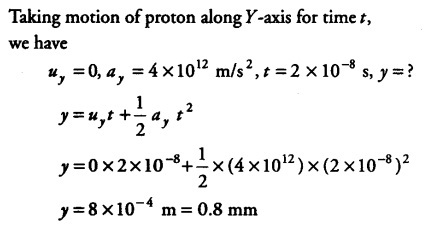# A proton is travelling with horizontal velocity

A proton is travelling with horizontal velocity \${ v }{ x }\$ = 2.5 x \${{10}^{8}}\$cm/s. Calculate the transverse deflection in travelling horizontal distance, x = 5 cm in electric field of
\${ E }
{ γ }\$ = 400 V/cm, mass of proton, m = 1.6 x \${{10}^{-24}}\$ g, charge on proton, e =1.6 x\${{10}^{-19}}\$ C.